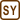## Lake Water Salting

### Task number: 1271

A spoonful of salt (ca. 2 g) was poured into a lake with an average depth of 10 m and area of 10 km2.

Provided that the salt dissolved evenly, determine how many sodium ions contains a spoonful of lake water (5 ml).

• #### Hint

Chemically, the table salt is sodium chloride, i.e. one „molecule“ of salt consists of one sodium atom and one chlorine atom .

First determine how many sodium atoms were poured into the lake and then determine how many of them there are in one spoonful of lake water.

• #### Numerical values

 h = 10 m the average lake depth S = 10 km2 = 107 m2 the water surface area m = 2 g = 2·10−3 kg the mass of salt VS = 5 ml = 5 cm3 = 5·10−6 m3 the volume of the spoon NS = ? the number of sodium ions in a spoonful we are looking for
• #### Analysis

Terminological note: The salt crystals, in fact, consist of sodium cations and chlorine anions held together by ionic bond. When the salt dissolves, the crystals disintegrate into individual ions. Therefore it is dubious to talk about a salt molecule. To simplify the matter we will use the term molecule of salt understanding couple of sodium and chlorine ions.

First we determine the number of salt molecules (NaCl) poured into the lake. As each molecule contains exactly one sodium ion, the number of sodium ions is equivalent to the number of salt molecules. Providing that the salt dissolved evenly we can determine the density of sodium ions in the lake water and thus the number of ions in a spoonful of water.

• #### Solution

The relative molecular mass of salt is

$M_r\mathrm{(NaCl)}\,=\,A_r\mathrm{(Na)} + A_r\mathrm{(Cl)}$ $M_r\mathrm{(NaCl)}\,=\,23.0 \,+\, 35.5 \,=\,58.5\,.$

We can find the number N of molecules by dividing the total mass of salt by the mass of a single molecule

$N\,=\,\frac{m}{m_0}\,=\,\frac{m}{M_r m_u}$ $N\,=\,\frac{2\,\cdot\,10^{-3}\mathrm{kg}}{58.5\,\cdot\,1.66\,\cdot\,10^{-27}\mathrm{kg}}\,\dot=\,2.06\,\cdot\,10^{22}$

Other way to calculate the number of molecules is to use the amount of substance n that can be determined by molar mass M.

$N\,=\,n N_A\,=\,\frac{m N_A}{M}\,=\,\frac{m N_A}{M_r \,\cdot\,10^{-3}\mathrm{kg\, mol}^{-1}}$ $N\,=\,\frac{2 \,\cdot\,10^{-3}\,\mathrm{kg}\,\cdot\,6.022\,\cdot\,10^{23}\,\mathrm{mol}^{-1}}{58.5 \,\cdot\,10^{-3}\,\mathrm{kg\, mol}^{-1}}\,\dot=\,2.06\,\cdot\,10^{22}$

The number of salt molecules is equal to the number of sodium ions.

The total volume of the lake water V is equal to the product of its surface area S and its average depth h.

$V\,=\,S h\,=\,10\,\cdot\,10^6 \,\mathrm{m}^{2}\,\cdot\,10 \,\mathrm{m}\,=\,10^8 \,\mathrm{m}^3.$

Assuming that salt dissolved evenly, the proportion of the number of sodium ions in the lake to the total lake volume must be equal to the proportion of the number of ions in a spoonful to the volume of the spoon. This is actually the bulk density of ions which has to be the same in the lake as in the spoonful.

$\frac{N}{V}\,=\,\frac{N_S}{V_S}$

Thus we can evaluate NS and we substitute the formerly derived general equations for N and V:

$N_S\,=\,\frac{N}{V}\,V_S\,=\,\frac{\frac{m\,N_A}{M}}{Sh}\,V_S\,=\,\frac{m\,N_A}{MSh}\,V_S$ $N_S\,=\,\frac{2\,\cdot\,10^{-3} \,\cdot\,6.022\,\cdot\,10^{23}}{58.5\,\cdot\,10^{-3}\,\cdot\,10^{7}\,\cdot\,10}\,\cdot\,5\,\cdot\,10^{-6}\,\dot=\,1\,\cdot\,10^{9}$
• #### Answer

Approximately a billion of sodium ions is in one spoonful of lake water. We can see that if we pour even a small quantity of salt into the large volume of water into the lake, relatively small volume of a spoon contains a huge number of particles.